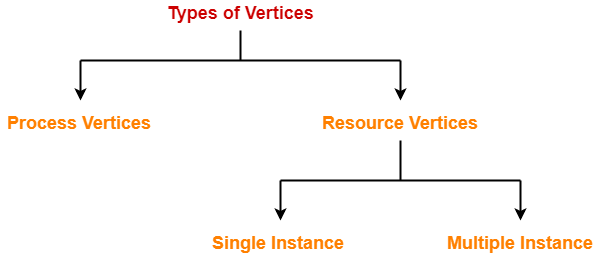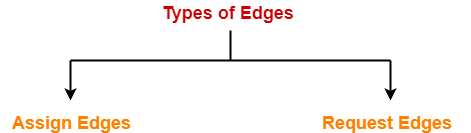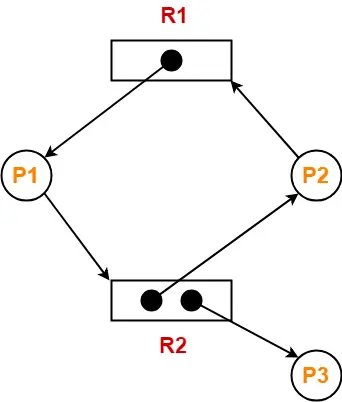# Resource Allocation Graph | Operating System

## Resource Allocation Graph-

 Resource Allocation Graph (RAG) is a graph that represents the state of a system pictorially.

It gives complete information about the state of a system such as-

• How many processes exist in the system?
• How many instances of each resource type exist?
• How many instances of each resource type are allocated?
• How many instances of each resource type are still available?
• How many instances of each resource type are held by each process?
• How many instances of each resource type does each process need for execution?

## Components Of RAG-

There are two major components of a Resource Allocation Graph-

1. Vertices
2. Edges

## Vertices-

There are following types of vertices in a Resource Allocation Graph-1. Process Vertices
2. Resource Vertices

## Process Vertices-

• Process vertices represent the processes.
• They are drawn as a circle by mentioning the name of process inside the circle.

## Resource Vertices-

• Resource vertices represent the resources.
• Depending on the number of instances that exists in the system, resource vertices may be single instance or multiple instance.
• They are drawn as a rectangle by mentioning the dots inside the rectangle.
• The number of dots inside the rectangle indicates the number of instances of that resource existing in the system.

## Edges-

There are two types of edges in a Resource Allocation Graph-1. Assign Edges
2. Request Edges

## Assign Edges-

• Assign edges represent the assignment of resources to the processes.
• They are drawn as an arrow where the head of the arrow points to the process and tail of the process points to the instance of the resource.

## Request Edges-

• Request edges represent the waiting state of processes for the resources.
• They are drawn as an arrow where the head of the arrow points to the instance of the resource and tail of the process points to the process.
• If a process requires ‘n’ instances of a resource type, then ‘n’ assign edges will be drawn.

## Example Of RAG-

The following diagram represents a Resource Allocation Graph-It gives the following information-

• There exist three processes in the system namely P1, P2 and P3.
• There exist two resources in the system namely R1 and R2.
• There exists a single instance of resource R1 and two instances of resource R2.
• Process P1 holds one instance of resource R1 and is waiting for an instance of resource R2.
• Process P2 holds one instance of resource R2 and is waiting for an instance of resource R1.
• Process P3 holds one instance of resource R2 and is not waiting for anything.

To gain better understanding about Resource Allocation Graph,

Watch this Video Lecture

Next Article- Practice Problems On Resource Allocation Graph

Get more notes and other study material of Operating System.

Watch video lectures by visiting our YouTube channel LearnVidFun.

SummaryArticle Name
Resource Allocation Graph | Operating System
Description
In OS, Resource Allocation Graph (RAG) is a graph that represents the state of a system pictorially. Whether the system is in a deadlock state or not can be predicted using Resource Allocation Graph.
Author
Publisher Name
Gate Vidyalay
Publisher Logo